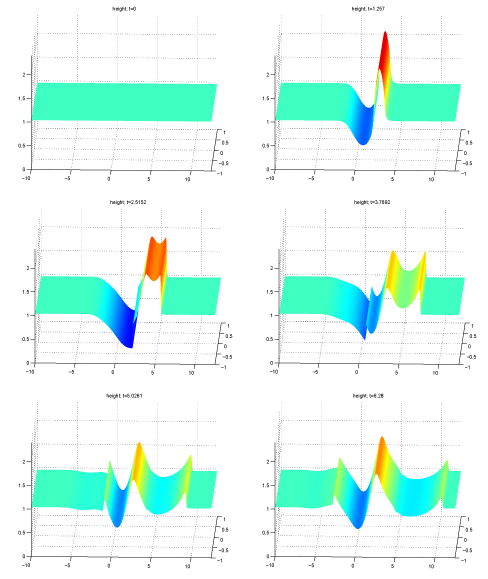# Numerical MathematicsThe research group "Numerical Analysis" covers the whole spectrum of numerical mathematics: from mathematical modelling, numerical analysis, to scientific computing.
In particularly, we focus on various problems arising from natural sciences and engineering. Some of the topics that are currently being studied include:

• inverse problems in geophysics
• electric impedance tomography
• computational fluid dynamics
• hyperbolic conservation laws
• adaptive numerical methods for partial differential equations
• mathematical biologyTime evolution of shallow water waves on a rotated plane. An important property is the development of discontinuous parts of the solution, so-called shocks, that are typical for hyperbolic conservation laws. The presented solution converges in time to a steady state, the so-called geostrophic equillibrium.